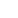Share

# UTKU'NUN BAŞINA BELA OLMAMA KALAN ZAMAN

15 August 2020 year at 09:00 PM

time left:

Bunu sen istedin :)))))))))))))))))))))))))))))))))

## THE MOST ACTIVE USERS

••••••